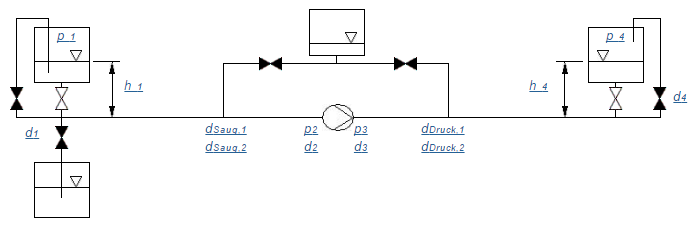﻿﻿﻿ Bernoulli ﻿ ﻿ ﻿

# BernoulliIn section BERNOULLI you calculate the changes in pressure caused by the frictionless fluid flow. Bernoulli´s equation

p + ρgh + ρ/2v2 = const.

takes into consideration the ambient pressure p, the hydrostatic pressure ρgh and the dynamic pressure ρ/2v2 in the frictionless flow.
Pressure losses caused by fluids viscosity (friction at the pipe wall, turbulence) can be calculated in section II. Piping and section III. Miscellaneous .

 p2 = p1 + ρ g h1 + ρ/2 (v12 - v22) Suction side p3 = p4 + ρ g h4 + ρ/2 (v42 - v32) Pressure side

 p1 : Absolute pressure above liquid level in the source tank. In case of a loop absolute pressure in the equalizing tank. p2 : Static pressure (gauge pressure as absolute pressure) at the suction side. p3 : Static pressure (gauge pressure as absolute pressure) at the discharge port. Diagrams of the system curves won´t be available, if p3 ≤ p2 (turbine). p4 : Absolute pressure above liquid level in target vessel. In case of a loop absolute pressure in the equalizing tank. ρ : Density g : Acceleration due to gravity, g = 9,81 m/s2 h1 : Distance between liquid level in the source tank (in case of a loop liquid level in the equalizing tank) and pipe center at the pump suction side. The value is < 0, if the liquid level of the source tank is below the suction side of the pump. h4 : Distance between lquid level in the target vessel (in case of a loop liquid level in the equalizing tank) and pipe center at the pump pressure side. v1, d1 : Average speed at the start cross section with diameter d1. If you didn´t choose a loop, TECCINESS will set d1 = ∞. This leads to v1 = 0. Pressure losses at the intake can be calculated in section III. Miscellaneous . In case of a loop v1 equals the speed at the outlet of the equalizing tank ( d1 = d4 ). v2, d2 : Average speed at the suction side with internal diameter d2 v3, d3 : Average speed at the pressure side with internal diameter d3 v4, d4 : Average speed at the final cross section with diameter d4. If you choose filling from below TECCINESS sets d4 = ∞ . This leads to v4 = 0. Pressure losses at the outlet can be calculated in section III. Miscellaneous . In case of a loop v4 equals the speed at the inlet of the equalizing tank ( d1 = d4 ).

﻿